Case Based Questions (MCQ)

Class 10
Chapter 10 Class 10 - Light - Reflection and Refraction

## Following figure illustrates the ray diagram for  the formation of images by a concave mirror. The  position of the object is beyond the centre of  curvature of the concave mirror. On the basis of the given diagram, answer any four questions from (a)  to (e).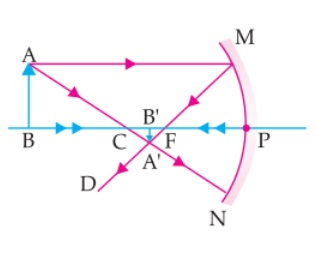### (iv) At 20 cm

Ans.

• As seen from the figure, the image formed is between focus and centre of curvature
• Thus, Image is formed between 10 and 20 cm.

So, the correct answer is (ii)

### (iv) positive, positive

Ans.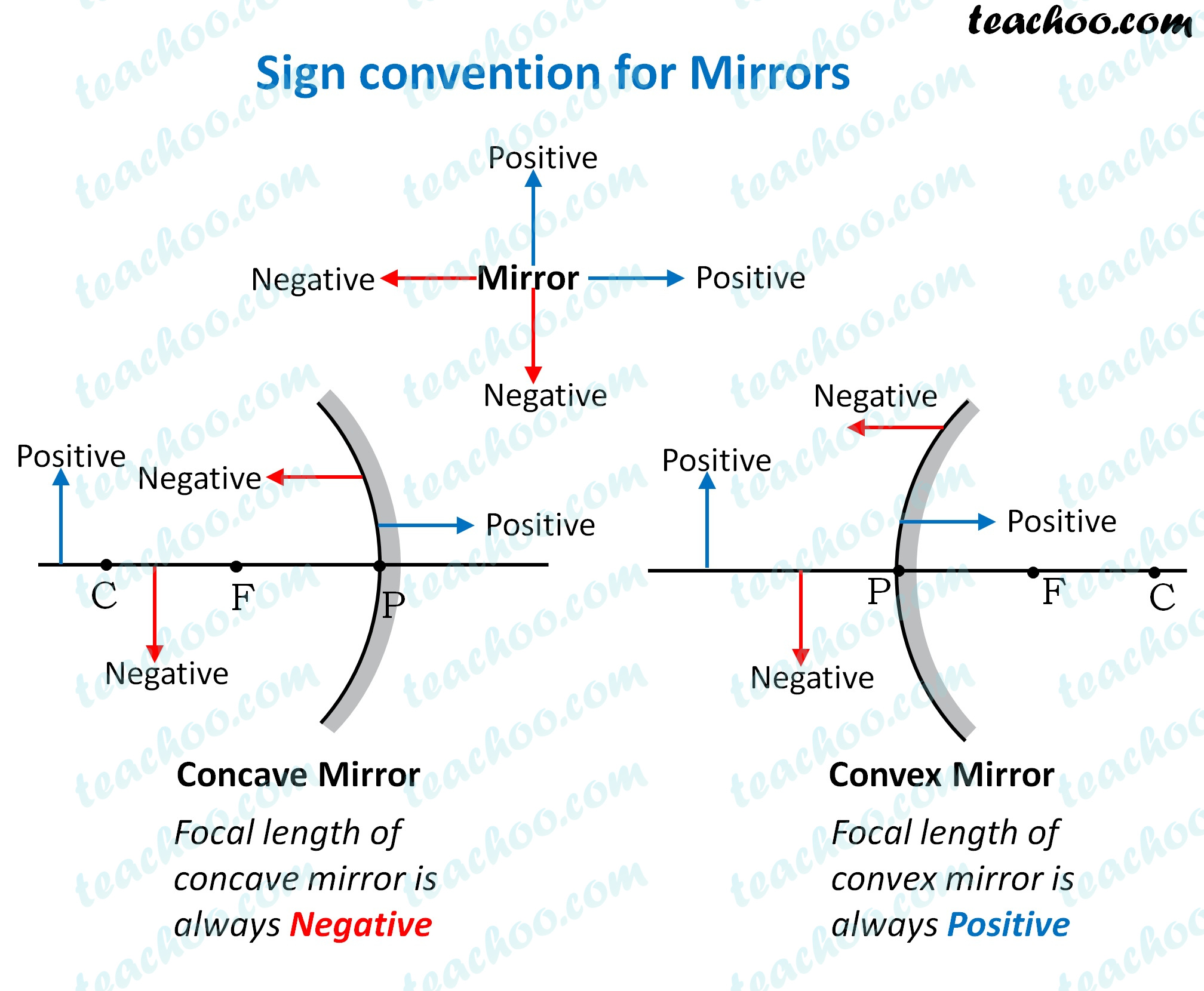According to the sign convention for concave mirror

•  The image distance is negative when the image is formed in front of the mirror
•  The image distance is positive when the image   is formed behind the mirror

So, the correct answer is (iii) - negative, positive

### (iv) 0.1

Ans.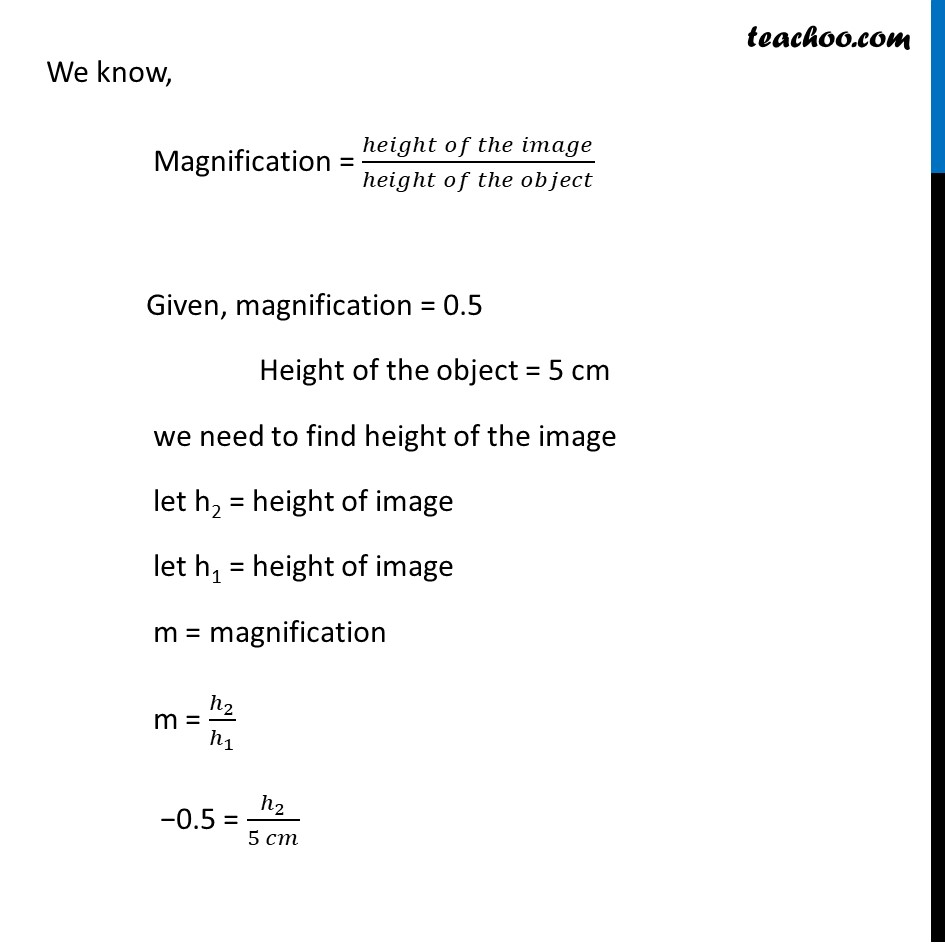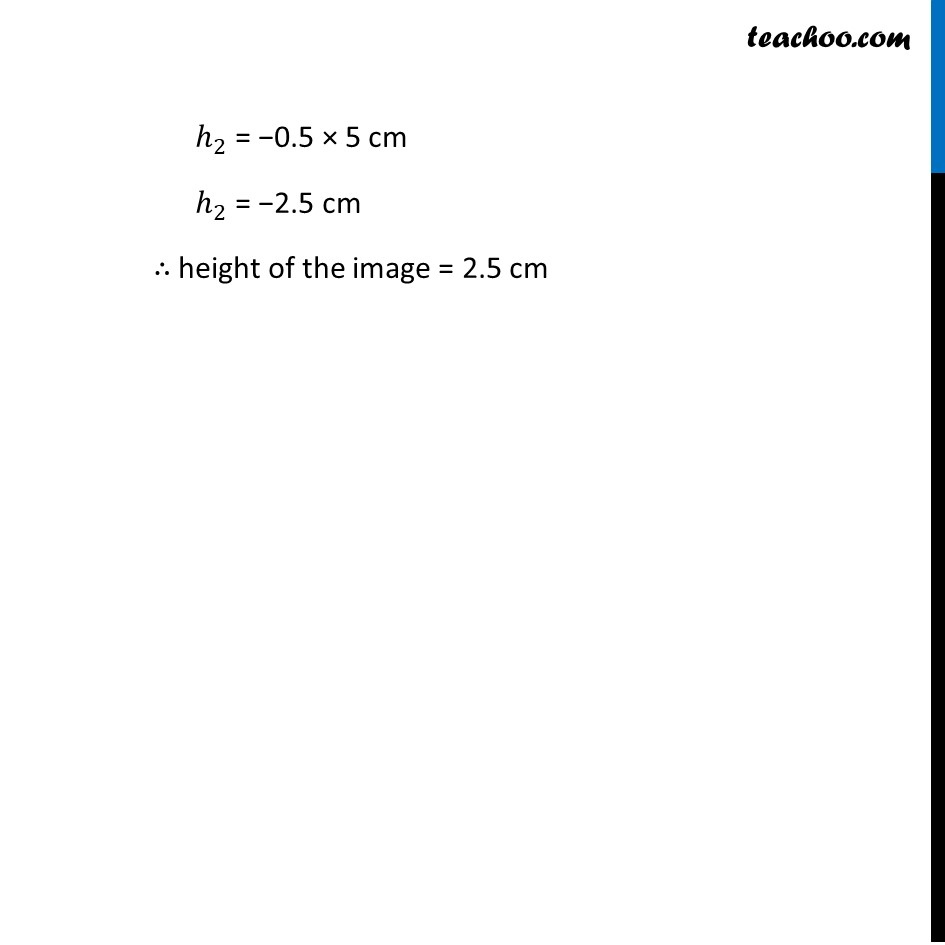So, the correct answer is  (i) – 2.5

### (iv) Virtual and inverted

Ans.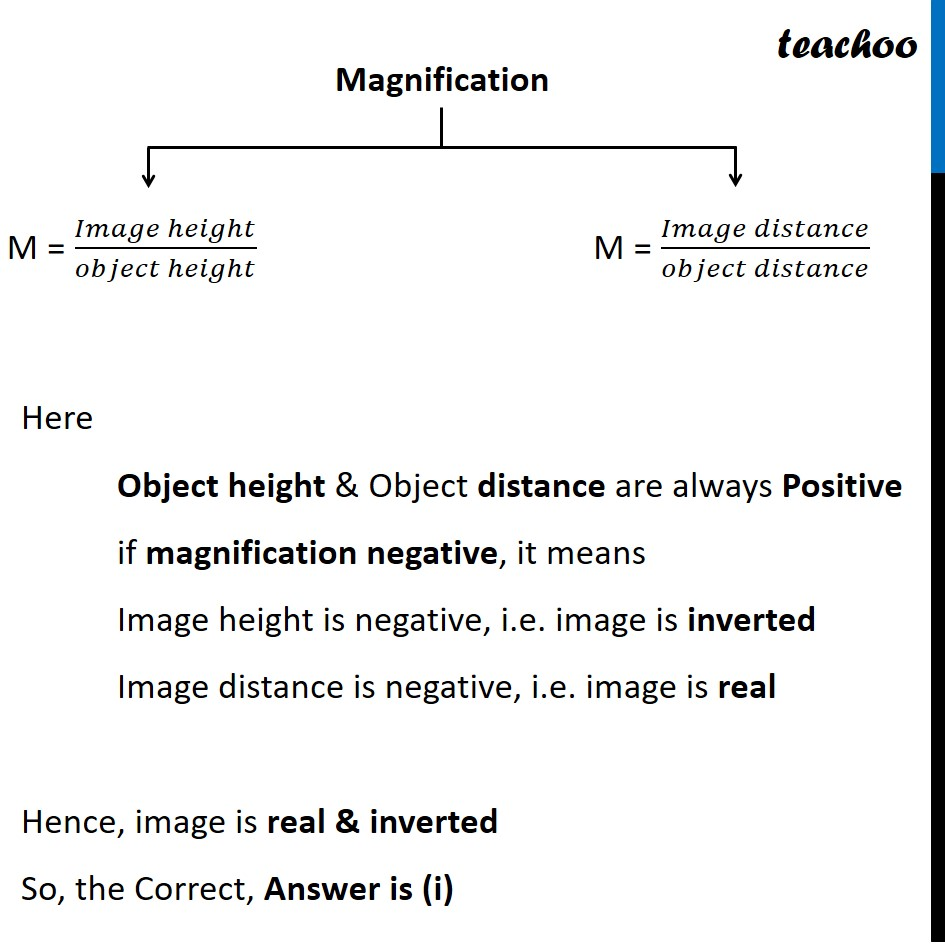So, the correct answer is (i) Real and inverted

### (iv) Between P and F

Ans.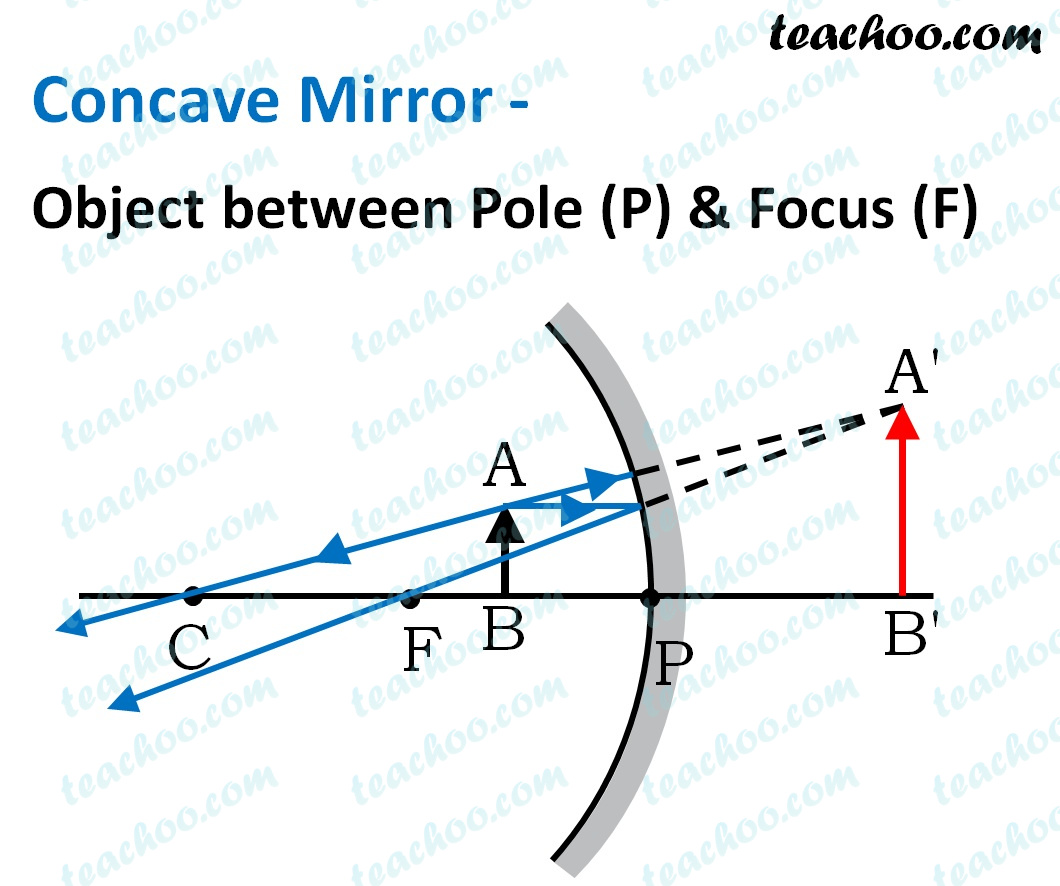From figure we note that:

• If we place Object between P ole and F ocus
• Concave mirror forms virtual and erect images.

So, the correct answer is (iv) = Between P and F

Learn in your speed, with individual attention - Teachoo Maths 1-on-1 Class